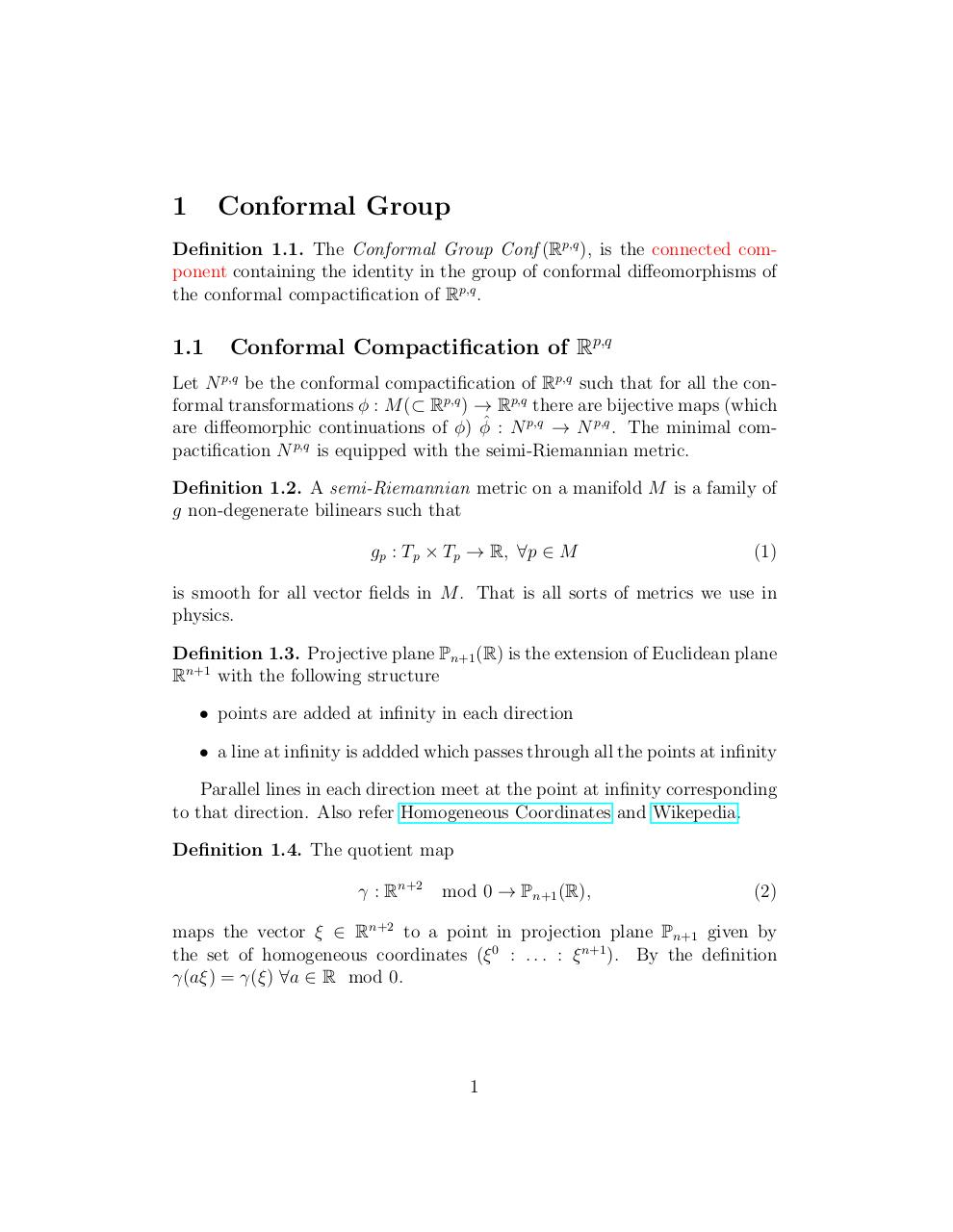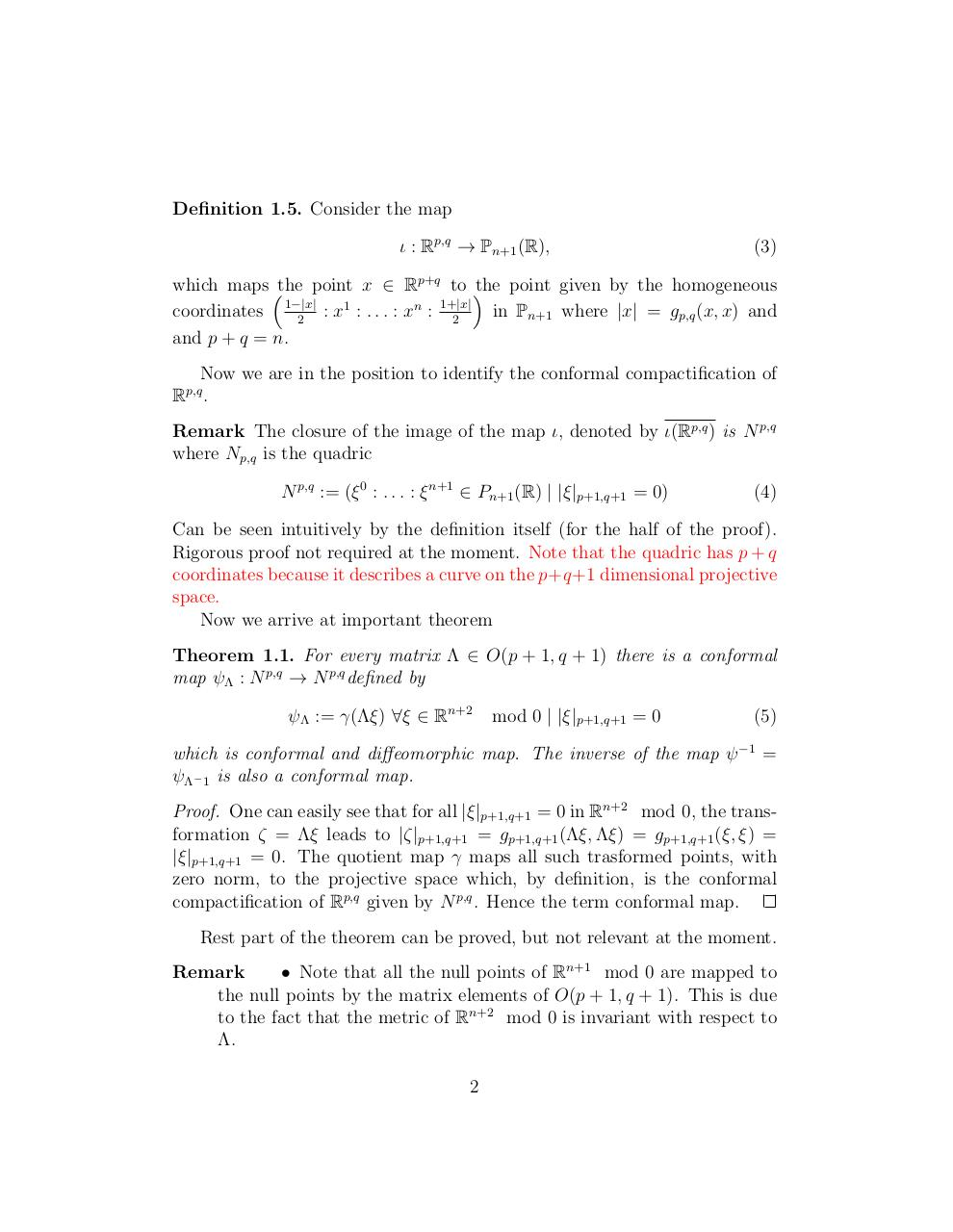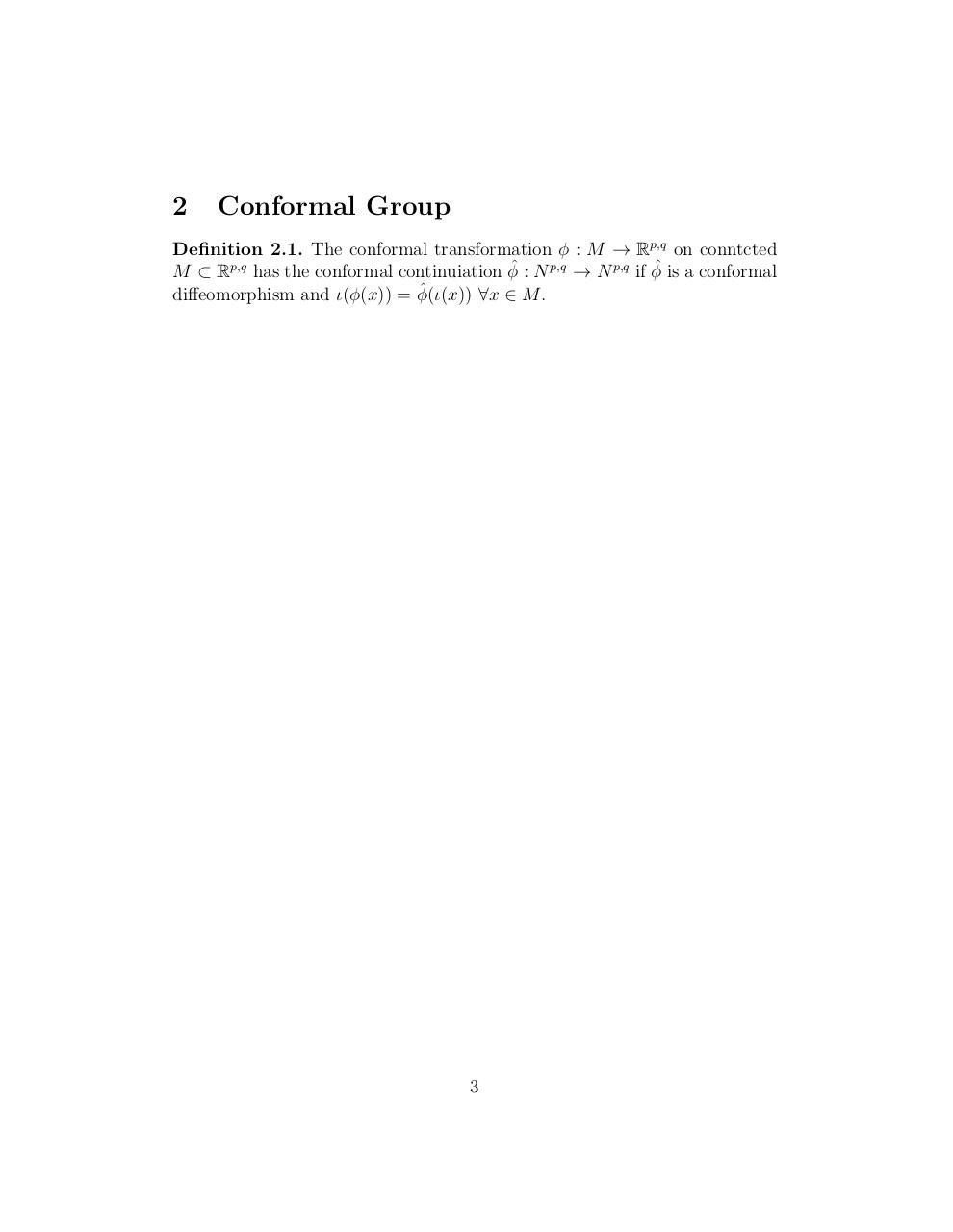# notes .pdf

### File information

Original filename: notes.pdf

This PDF 1.5 document has been generated by LaTeX with hyperref package / pdfTeX-1.40.16, and has been sent on pdf-archive.com on 23/01/2016 at 14:18, from IP address 72.182.x.x. The current document download page has been viewed 621 times.
File size: 117 KB (3 pages).
Privacy: public file

notes.pdf (PDF, 117 KB)

### Document preview

1

Conformal Group

Definition 1.1. The Conformal Group Conf (Rp,q ), is the connected component containing the identity in the group of conformal diffeomorphisms of
the conformal compactification of Rp,q .

1.1

Conformal Compactification of Rp,q

Let N p,q be the conformal compactification of Rp,q such that for all the conformal transformations φ : M (⊂ Rp,q ) → Rp,q there are bijective maps (which
are diffeomorphic continuations of φ) φˆ : N p,q → N p,q . The minimal compactification N p,q is equipped with the seimi-Riemannian metric.
Definition 1.2. A semi-Riemannian metric on a manifold M is a family of
g non-degenerate bilinears such that
gp : Tp × Tp → R, ∀p ∈ M

(1)

is smooth for all vector fields in M . That is all sorts of metrics we use in
physics.
Definition 1.3. Projective plane Pn+1 (R) is the extension of Euclidean plane
Rn+1 with the following structure
• points are added at infinity in each direction
• a line at infinity is addded which passes through all the points at infinity
Parallel lines in each direction meet at the point at infinity corresponding
to that direction. Also refer Homogeneous Coordinates and Wikepedia.
Definition 1.4. The quotient map
γ : Rn+2

mod 0 → Pn+1 (R),

(2)

maps the vector ξ ∈ Rn+2 to a point in projection plane Pn+1 given by
the set of homogeneous coordinates (ξ 0 : . . . : ξ n+1 ). By the definition
γ(aξ) = γ(ξ) ∀a ∈ R mod 0.

1

Definition 1.5. Consider the map
ι : Rp,q → Pn+1 (R),

(3)

which maps the point x ∈ Rp+q to the point given by the homogeneous
: x1 : . . . : xn : 1+|x|
in Pn+1 where |x| = gp,q (x, x) and
coordinates 1−|x|
2
2
and p + q = n.
Now we are in the position to identify the conformal compactification of
R .
p,q

Remark The closure of the image of the map ι, denoted by ι(Rp,q ) is N p,q
N p,q := (ξ 0 : . . . : ξ n+1 ∈ Pn+1 (R) | |ξ|p+1,q+1 = 0)

(4)

Can be seen intuitively by the definition itself (for the half of the proof).
Rigorous proof not required at the moment. Note that the quadric has p + q
coordinates because it describes a curve on the p+q+1 dimensional projective
space.
Now we arrive at important theorem
Theorem 1.1. For every matrix Λ ∈ O(p + 1, q + 1) there is a conformal
map ψΛ : N p,q → N p,q defined by
ψΛ := γ(Λξ) ∀ξ ∈ Rn+2

mod 0 | |ξ|p+1,q+1 = 0

(5)

which is conformal and diffeomorphic map. The inverse of the map ψ −1 =
ψΛ− 1 is also a conformal map.
Proof. One can easily see that for all |ξ|p+1,q+1 = 0 in Rn+2 mod 0, the transformation ζ = Λξ leads to |ζ|p+1,q+1 = gp+1,q+1 (Λξ, Λξ) = gp+1,q+1 (ξ, ξ) =
|ξ|p+1,q+1 = 0. The quotient map γ maps all such trasformed points, with
zero norm, to the projective space which, by definition, is the conformal
compactification of Rp,q given by N p,q . Hence the term conformal map.
Rest part of the theorem can be proved, but not relevant at the moment.
Remark
• Note that all the null points of Rn+1 mod 0 are mapped to
the null points by the matrix elements of O(p + 1, q + 1). This is due
to the fact that the metric of Rn+2 mod 0 is invariant with respect to
Λ.
2

2

Conformal Group

Definition 2.1. The conformal transformation φ : M → Rp,q on conntcted
M ⊂ Rp,q has the conformal continuiation φˆ : N p,q → N p,q if φˆ is a conformal
ˆ
diffeomorphism and ι(φ(x)) = φ(ι(x))
∀x ∈ M .

3#### HTML Code

Copy the following HTML code to share your document on a Website or Blog

#### QR Code### Related keywords